## A 20.0 kgkg rock is sliding on a rough, horizontal surface at 8.00 m/sm/s and eventually stops due to friction. The coefficient of kinetic f

Question

A 20.0 kgkg rock is sliding on a rough, horizontal surface at 8.00 m/sm/s and eventually stops due to friction. The coefficient of kinetic friction between the rock and the surface is 0.200.What average power is produced by friction as the rock stops?

in progress 0
3 weeks 2021-08-26T04:58:09+00:00 1 Answers 0 views 0

The average power is 157W.

Explanation:

To calculate the average power produced by friction we need the work done by that force, and the time the rock takes to stop. To calculate the work, we first have to calculate the magnitude of the frictional force. In order to do that, we first write the equations of motion of the rock in the horizontal and the vertical axes: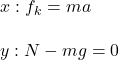Since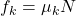, we have: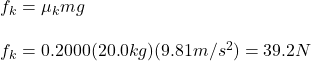And the acceleration is: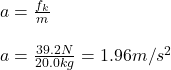Next, we need the distance traveled by the rock. To obtain that, we use the kinematics equation: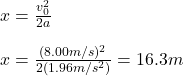Since the displacement and the frictional force have opposite directions, the work must be negative. So, the work done by the friction is: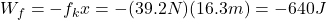Now, we still need the time the rock takes to stop. Then, we use the kinematics equation: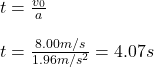Finally, using the definition of average power, we have: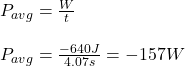This means that the average power produced by friction, as the rock stops, is 157W. The negative sign means that the frictional force does a negative work on the rock; it absorbs its energy instead of giving it more energy.